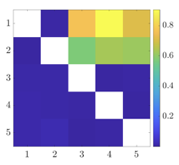# Transfer entropy for network reconstruction in a simple dynamical model### Abstract

Dynamics on a network are ubiquitous in areas as diverse as genetics, meteorology, and the social sciences. By a network, we simply mean a system of interacting agents. A common problem is to reconstruct a network given the behavior of its agents, that is, given a set of time series describing the states of each node on the network, determine which other nodes are influencing that node. Information theory provides one set of tools for doing so in a model-independent manner. A popular tool in this game is called transfer entropy. Transfer entropy measures the reduction in uncertainty in predicting the state of one agent at a time (t+1) from its state at time t given the additional knowledge of the state of a second j at time t, compared with predicting it the knowledge of the first agent alone. This can be thought of as measuring the transfer of information from the second agent to the first. We analyze a simple model in which we derive asymptotic formulae relating the transfer entropy between two nodes to the strength of the connection between the nodes. Higher-order terms in this formula show the subtle effect of network topology on the transfer entropy. This allows for more accurate network reconstruction in this particular model and points to possible sources of errors when transfer entropy is used to reconstruct networks in a more general setting.

Date
Feb 14, 2020 11:40 AM — 12:40 PM
Location
New Jersey Institute of Technology
Newark, NJ##### Roy Goodman
###### Associate Professor, Department of Mathematical Sciences

My research interests include dynamical systems and nonlinear waves, vortex dynamics, quantum graphs, and network inference